x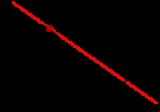DerivativeOverview

In calculus
Calculus
Calculus is a branch of mathematics focused on limits, functions, derivatives, integrals, and infinite series. This subject constitutes a major part of modern mathematics education. It has two major branches, differential calculus and integral calculus, which are related by the fundamental theorem...

, a branch of mathematics
Mathematics
Mathematics is the study of quantity, space, structure, and change. Mathematicians seek out patterns and formulate new conjectures. Mathematicians resolve the truth or falsity of conjectures by mathematical proofs, which are arguments sufficient to convince other mathematicians of their validity...

, the derivative is a measure of how a function
Function (mathematics)
In mathematics, a function associates one quantity, the argument of the function, also known as the input, with another quantity, the value of the function, also known as the output. A function assigns exactly one output to each input. The argument and the value may be real numbers, but they can...

changes as its input changes. Loosely speaking, a derivative can be thought of as how much one quantity is changing in response to changes in some other quantity; for example, the derivative of the position of a moving object with respect to time is the object's instantaneous velocity
Velocity
In physics, velocity is speed in a given direction. Speed describes only how fast an object is moving, whereas velocity gives both the speed and direction of the object's motion. To have a constant velocity, an object must have a constant speed and motion in a constant direction. Constant ...

.

The derivative of a function at a chosen input value describes the best linear approximation
Linear approximation
In mathematics, a linear approximation is an approximation of a general function using a linear function . They are widely used in the method of finite differences to produce first order methods for solving or approximating solutions to equations.-Definition:Given a twice continuously...

of the function near that input value.Unanswered Questionsfind nth derivative of: 1) sin^3xcos^3x 2) cos^4xEncyclopedia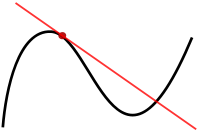In calculus
Calculus
Calculus is a branch of mathematics focused on limits, functions, derivatives, integrals, and infinite series. This subject constitutes a major part of modern mathematics education. It has two major branches, differential calculus and integral calculus, which are related by the fundamental theorem...

, a branch of mathematics
Mathematics
Mathematics is the study of quantity, space, structure, and change. Mathematicians seek out patterns and formulate new conjectures. Mathematicians resolve the truth or falsity of conjectures by mathematical proofs, which are arguments sufficient to convince other mathematicians of their validity...

, the derivative is a measure of how a function
Function (mathematics)
In mathematics, a function associates one quantity, the argument of the function, also known as the input, with another quantity, the value of the function, also known as the output. A function assigns exactly one output to each input. The argument and the value may be real numbers, but they can...

changes as its input changes. Loosely speaking, a derivative can be thought of as how much one quantity is changing in response to changes in some other quantity; for example, the derivative of the position of a moving object with respect to time is the object's instantaneous velocity
Velocity
In physics, velocity is speed in a given direction. Speed describes only how fast an object is moving, whereas velocity gives both the speed and direction of the object's motion. To have a constant velocity, an object must have a constant speed and motion in a constant direction. Constant ...

.

The derivative of a function at a chosen input value describes the best linear approximation
Linear approximation
In mathematics, a linear approximation is an approximation of a general function using a linear function . They are widely used in the method of finite differences to produce first order methods for solving or approximating solutions to equations.-Definition:Given a twice continuously...

of the function near that input value. For a real-valued function
Real-valued function
In mathematics, a real-valued function is a function that associates to every element of the domain a real number in the image....

of a single real variable, the derivative at a point equals the slope
Slope
In mathematics, the slope or gradient of a line describes its steepness, incline, or grade. A higher slope value indicates a steeper incline....

of the tangent line
Tangent
In geometry, the tangent line to a plane curve at a given point is the straight line that "just touches" the curve at that point. More precisely, a straight line is said to be a tangent of a curve at a point on the curve if the line passes through the point on the curve and has slope where f...

to the graph of the function
Graph of a function
In mathematics, the graph of a function f is the collection of all ordered pairs . In particular, if x is a real number, graph means the graphical representation of this collection, in the form of a curve on a Cartesian plane, together with Cartesian axes, etc. Graphing on a Cartesian plane is...

at that point. In higher dimensions, the derivative of a function at a point is a linear transformation
Linear transformation
In mathematics, a linear map, linear mapping, linear transformation, or linear operator is a function between two vector spaces that preserves the operations of vector addition and scalar multiplication. As a result, it always maps straight lines to straight lines or 0...

called the linearization
Linearization
In mathematics and its applications, linearization refers to finding the linear approximation to a function at a given point. In the study of dynamical systems, linearization is a method for assessing the local stability of an equilibrium point of a system of nonlinear differential equations or...

. A closely related notion is the differential of a function
Differential of a function
In calculus, the differential represents the principal part of the change in a function y = ƒ with respect to changes in the independent variable. The differential dy is defined bydy = f'\,dx,...

.

The process of finding a derivative is called differentiation. The reverse process is called antidifferentiation
Antiderivative
In calculus, an "anti-derivative", antiderivative, primitive integral or indefinite integralof a function f is a function F whose derivative is equal to f, i.e., F ′ = f...

. The fundamental theorem of calculus
Fundamental theorem of calculus
The first part of the theorem, sometimes called the first fundamental theorem of calculus, shows that an indefinite integration can be reversed by a differentiation...

states that antidifferentiation is the same as integration
Integral
Integration is an important concept in mathematics and, together with its inverse, differentiation, is one of the two main operations in calculus...

. Differentiation and integration constitute the two fundamental operations in single-variable calculus.

## Differentiation and the derivative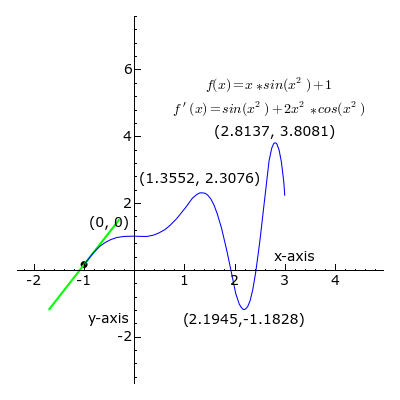Differentiation is a method to compute the rate at which a dependent output y changes with respect to the change in the independent input x. This rate of change is called the derivative of y with respect to x. In more precise language, the dependence of y upon x means that y is a function
Function (mathematics)
In mathematics, a function associates one quantity, the argument of the function, also known as the input, with another quantity, the value of the function, also known as the output. A function assigns exactly one output to each input. The argument and the value may be real numbers, but they can...

of x. This functional relationship is often denoted y = ƒ(x), where ƒ denotes the function. If x and y are real number
Real number
In mathematics, a real number is a value that represents a quantity along a continuum, such as -5 , 4/3 , 8.6 , √2 and π...

s, and if the graph
Graph of a function
In mathematics, the graph of a function f is the collection of all ordered pairs . In particular, if x is a real number, graph means the graphical representation of this collection, in the form of a curve on a Cartesian plane, together with Cartesian axes, etc. Graphing on a Cartesian plane is...

of y is plotted against x, the derivative measures the slope
Slope
In mathematics, the slope or gradient of a line describes its steepness, incline, or grade. A higher slope value indicates a steeper incline....

of this graph at each point.

The simplest case is when y is a linear function
Linear function
In mathematics, the term linear function can refer to either of two different but related concepts:* a first-degree polynomial function of one variable;* a map between two vector spaces that preserves vector addition and scalar multiplication....

of x, meaning that the graph of y against x is a straight line. In this case, y = ƒ(x) = m x + b, for real numbers m and b, and the slope m is given by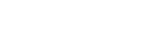where the symbol Δ (the uppercase form of the Greek letter Delta
Delta (letter)
Delta is the fourth letter of the Greek alphabet. In the system of Greek numerals it has a value of 4. It was derived from the Phoenician letter Dalet...

) is an abbreviation for "change in." This formula is true because
y + Δy = ƒ(x+ Δx) = m (x + Δx) + b = m x + b + m Δx = y + mΔx.

It follows that Δy = m Δx.

This gives an exact value for the slope of a straight line.
If the function ƒ is not linear (i.e. its graph is not a straight line), however, then the change in y divided by the change in x varies: differentiation is a method to find an exact value for this rate of change at any given value of x.
The idea, illustrated by Figures 1-3, is to compute the rate of change as the limiting value
Limit of a function
In mathematics, the limit of a function is a fundamental concept in calculus and analysis concerning the behavior of that function near a particular input....

of the ratio of the differences
Difference quotient
The primary vehicle of calculus and other higher mathematics is the function. Its "input value" is its argument, usually a point expressible on a graph...

Δy / Δx as Δx becomes infinitely small.

In Leibniz's notation, such an infinitesimal
Infinitesimal
Infinitesimals have been used to express the idea of objects so small that there is no way to see them or to measure them. The word infinitesimal comes from a 17th century Modern Latin coinage infinitesimus, which originally referred to the "infinite-th" item in a series.In common speech, an...

change in x is denoted by dx, and the derivative of y with respect to x is written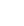suggesting the ratio of two infinitesimal quantities. (The above expression is read as "the derivative of y with respect to x", "d y by d x", or "d y over d x". The oral form "d y d x" is often used conversationally, although it may lead to confusion.)

The most common approach to turn this intuitive idea into a precise definition uses limits, but there are other methods, such as non-standard analysis
Non-standard analysis
Non-standard analysis is a branch of mathematics that formulates analysis using a rigorous notion of an infinitesimal number.Non-standard analysis was introduced in the early 1960s by the mathematician Abraham Robinson. He wrote:...

.

### Definition via difference quotients

Let ƒ be a real valued function. In classical geometry, the tangent line to the graph of the function ƒ at a real number a was the unique line through the point (a, ƒ(a)) that did not meet the graph of ƒ transversally, meaning that the line did not pass straight through the graph. The derivative of y with respect to x at a is, geometrically, the slope of the tangent line to the graph of ƒ at a. The slope of the tangent line is very close to the slope of the line through (a, ƒ(a)) and a nearby point on the graph, for example . These lines are called secant line
Secant line
A secant line of a curve is a line that intersects two points on the curve. The word secant comes from the Latin secare, to cut.It can be used to approximate the tangent to a curve, at some point P...

s. A value of h close to zero gives a good approximation to the slope of the tangent line, and smaller values (in absolute value
Absolute value
In mathematics, the absolute value |a| of a real number a is the numerical value of a without regard to its sign. So, for example, the absolute value of 3 is 3, and the absolute value of -3 is also 3...

) of h will, in general, give better approximation
Approximation
An approximation is a representation of something that is not exact, but still close enough to be useful. Although approximation is most often applied to numbers, it is also frequently applied to such things as mathematical functions, shapes, and physical laws.Approximations may be used because...

s. The slope m of the secant line is the difference between the y values of these points divided by the difference between the x values, that is,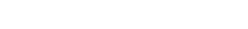This expression is Newton
Isaac Newton
Sir Isaac Newton PRS was an English physicist, mathematician, astronomer, natural philosopher, alchemist, and theologian, who has been "considered by many to be the greatest and most influential scientist who ever lived."...

's difference quotient
Difference quotient
The primary vehicle of calculus and other higher mathematics is the function. Its "input value" is its argument, usually a point expressible on a graph...

. The derivative is the value of the difference quotient as the secant lines approach the tangent line. Formally, the derivative of the function ƒ at a is the limit
Limit of a function
In mathematics, the limit of a function is a fundamental concept in calculus and analysis concerning the behavior of that function near a particular input....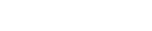of the difference quotient as h approaches zero, if this limit exists. If the limit exists, then ƒ is differentiable
Differentiable function
In calculus , a differentiable function is a function whose derivative exists at each point in its domain. The graph of a differentiable function must have a non-vertical tangent line at each point in its domain...

at a. Here ƒ′ (a) is one of several common notations for the derivative (see below).

Equivalently, the derivative satisfies the property that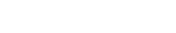which has the intuitive interpretation (see Figure 1) that the tangent line to ƒ at a gives the best linear
Linear
In mathematics, a linear map or function f is a function which satisfies the following two properties:* Additivity : f = f + f...

approximation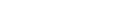to ƒ near a (i.e., for small h). This interpretation is the easiest to generalize to other settings (see below).

Substituting 0 for h in the difference quotient causes division by zero
Division by zero
In mathematics, division by zero is division where the divisor is zero. Such a division can be formally expressed as a / 0 where a is the dividend . Whether this expression can be assigned a well-defined value depends upon the mathematical setting...

, so the slope of the tangent line cannot be found directly using this method. Instead, define Q(h) to be the difference quotient as a function of h: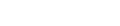Q(h) is the slope of the secant line between (a, ƒ(a)) and (a + h, ƒ(a + h)). If ƒ is a continuous function
Continuous function
In mathematics, a continuous function is a function for which, intuitively, "small" changes in the input result in "small" changes in the output. Otherwise, a function is said to be "discontinuous". A continuous function with a continuous inverse function is called "bicontinuous".Continuity of...

, meaning that its graph is an unbroken curve with no gaps, then Q is a continuous function away from h = 0. If the limit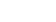exists, meaning that there is a way of choosing a value for Q(0) that makes the graph of Q a continuous function, then the function ƒ is differentiable at a, and its derivative at a equals Q(0).

In practice, the existence of a continuous extension of the difference quotient Q(h) to h = 0 is shown by modifying the numerator to cancel h in the denominator. This process can be long and tedious for complicated functions, and many shortcuts are commonly used to simplify the process.

### Example

The squaring function ƒ(x) = x² is differentiable at x = 3, and its derivative there is 6. This result is established by calculating the limit as h approaches zero of the difference quotient of ƒ(3):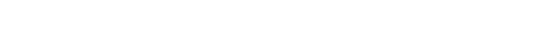The last expression shows that the difference quotient equals 6 + h when h ≠ 0 and is undefined when h = 0, because of the definition of the difference quotient. However, the definition of the limit says the difference quotient does not need to be defined when h = 0. The limit is the result of letting h go to zero, meaning it is the value that tends to as h becomes very small: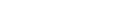Hence the slope of the graph of the squaring function at the point (3, 9) is 6, and so its derivative at x = 3 is ƒ '(3) = 6.

More generally, a similar computation shows that the derivative of the squaring function at x = a is ƒ '(a) = 2a.

### Continuity and differentiability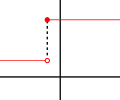If y = ƒ(x) is differentiable
Differentiable function
In calculus , a differentiable function is a function whose derivative exists at each point in its domain. The graph of a differentiable function must have a non-vertical tangent line at each point in its domain...

at a, then ƒ must also be continuous
Continuous function
In mathematics, a continuous function is a function for which, intuitively, "small" changes in the input result in "small" changes in the output. Otherwise, a function is said to be "discontinuous". A continuous function with a continuous inverse function is called "bicontinuous".Continuity of...

at a. As an example, choose a point a and let ƒ be the step function
Step function
In mathematics, a function on the real numbers is called a step function if it can be written as a finite linear combination of indicator functions of intervals...

that returns a value, say 1, for all x less than a, and returns a different value, say 10, for all x greater than or equal to a. ƒ cannot have a derivative at a. If h is negative, then a + h is on the low part of the step, so the secant line from a to a + h is very steep, and as h tends to zero the slope tends to infinity. If h is positive, then a + h is on the high part of the step, so the secant line from a to a + h has slope zero. Consequently the secant lines do not approach any single slope, so the limit of the difference quotient does not exist.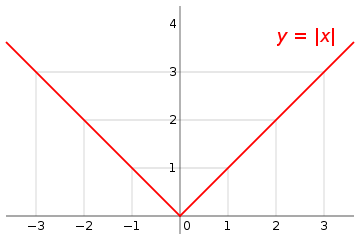However, even if a function is continuous at a point, it may not be differentiable there. For example, the absolute value
Absolute value
In mathematics, the absolute value |a| of a real number a is the numerical value of a without regard to its sign. So, for example, the absolute value of 3 is 3, and the absolute value of -3 is also 3...

function y = |x| is continuous at x = 0, but it is not differentiable there. If h is positive, then the slope of the secant line from 0 to h is one, whereas if h is negative, then the slope of the secant line from 0 to h is negative one. This can be seen graphically as a "kink" or a "cusp" in the graph at x = 0. Even a function with a smooth graph is not differentiable at a point where its tangent is vertical
Vertical tangent
In mathematics, a vertical tangent is tangent line that is vertical. Because a vertical line has infinite slope, a function whose graph has a vertical tangent is not differentiable at the point of tangency.- Limit definition :...

: For instance, the function y = 3x is not differentiable at x = 0.

In summary: for a function ƒ to have a derivative it is necessary
Necessary and sufficient conditions
In logic, the words necessity and sufficiency refer to the implicational relationships between statements. The assertion that one statement is a necessary and sufficient condition of another means that the former statement is true if and only if the latter is true.-Definitions:A necessary condition...

for the function ƒ to be continuous, but continuity alone is not sufficient
Necessary and sufficient conditions
In logic, the words necessity and sufficiency refer to the implicational relationships between statements. The assertion that one statement is a necessary and sufficient condition of another means that the former statement is true if and only if the latter is true.-Definitions:A necessary condition...

.

Most functions that occur in practice have derivatives at all points or at almost every
Almost everywhere
In measure theory , a property holds almost everywhere if the set of elements for which the property does not hold is a null set, that is, a set of measure zero . In cases where the measure is not complete, it is sufficient that the set is contained within a set of measure zero...

point. Early in the history of calculus, many mathematicians assumed that a continuous function was differentiable at most points. Under mild conditions, for example if the function is a monotone function or a Lipschitz function, this is true. However, in 1872 Weierstrass found the first example of a function that is continuous everywhere but differentiable nowhere. This example is now known as the Weierstrass function
Weierstrass function
In mathematics, the Weierstrass function is a pathological example of a real-valued function on the real line. The function has the property that it is continuous everywhere but differentiable nowhere...

. In 1931, Stefan Banach
Stefan Banach
Stefan Banach was a Polish mathematician who worked in interwar Poland and in Soviet Ukraine. He is generally considered to have been one of the 20th century's most important and influential mathematicians....

proved that the set of functions that have a derivative at some point is a meager set in the space of all continuous functions. Informally, this means that hardly any continuous functions have a derivative at even one point.

### The derivative as a function

Let ƒ be a function that has a derivative at every point a in the domain
Domain (mathematics)
In mathematics, the domain of definition or simply the domain of a function is the set of "input" or argument values for which the function is defined...

of ƒ. Because every point a has a derivative, there is a function that sends the point a to the derivative of ƒ at a. This function is written f′(x) and is called the derivative function or the derivative of ƒ. The derivative of ƒ collects all the derivatives of ƒ at all the points in the domain of ƒ.

Sometimes ƒ has a derivative at most, but not all, points of its domain. The function whose value at a equals f′(a) whenever f′(a) is defined and elsewhere is undefined is also called the derivative of ƒ. It is still a function, but its domain is strictly smaller than the domain of ƒ.

Using this idea, differentiation becomes a function of functions: The derivative is an operator whose domain is the set of all functions that have derivatives at every point of their domain and whose range is a set of functions. If we denote this operator by D, then D(ƒ) is the function f′(x). Since D(ƒ) is a function, it can be evaluated at a point a. By the definition of the derivative function, D(ƒ)(a) = f′(a).

For comparison, consider the doubling function ƒ(x) =2x; ƒ is a real-valued function of a real number, meaning that it takes numbers as inputs and has numbers as outputs: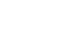The operator D, however, is not defined on individual numbers. It is only defined on functions: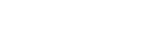Because the output of D is a function, the output of D can be evaluated at a point. For instance, when D is applied to the squaring function,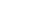D outputs the doubling function,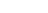which we named ƒ(x). This output function can then be evaluated to get ƒ(1) = 2, ƒ(2) = 4, and so on.

### Higher derivatives

Let ƒ be a differentiable function, and let f′(x) be its derivative. The derivative of (if it has one) is written and is called the second derivative
Second derivative
In calculus, the second derivative of a function ƒ is the derivative of the derivative of ƒ. Roughly speaking, the second derivative measures how the rate of change of a quantity is itself changing; for example, the second derivative of the position of a vehicle with respect to time is...

of ƒ. Similarly, the derivative of a second derivative, if it exists, is written and is called the
third derivative of ƒ. These repeated derivatives are called higher-order derivatives.

If x(t) represents the position of an object at time t, then the higher-order derivatives of x have physical interpretations. The second derivative of x is the derivative of x′(t), the velocity, and by definition this is the object's acceleration
Acceleration
In physics, acceleration is the rate of change of velocity with time. In one dimension, acceleration is the rate at which something speeds up or slows down. However, since velocity is a vector, acceleration describes the rate of change of both the magnitude and the direction of velocity. ...

. The third derivative of x is defined to be the jerk, and the fourth derivative is defined to be the jounce.

A function ƒ need not have a derivative, for example, if it is not continuous. Similarly, even if ƒ does have a derivative, it may not have a second derivative. For example, let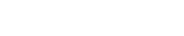Calculation shows that ƒ is a differentiable function whose derivative is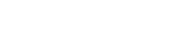is twice the absolute value function, and it does not have a derivative at zero. Similar examples show that a function can have k derivatives for any non-negative integer k but no (k + 1)-order derivative. A function that has k successive derivatives is called k times differentiable. If in addition the kth derivative is continuous, then the function is said to be of differentiability class Ck. (This is a stronger condition than having k derivatives. For an example, see differentiability class.) A function that has infinitely many derivatives is called infinitely differentiable or smooth
Smooth function
In mathematical analysis, a differentiability class is a classification of functions according to the properties of their derivatives. Higher order differentiability classes correspond to the existence of more derivatives. Functions that have derivatives of all orders are called smooth.Most of...

.

On the real line, every polynomial function is infinitely differentiable. By standard differentiation rules
Differentiation rules
This article is a summary of the differentiation rules in elementary calculus, i.e. rules for computing the derivative of a function in calculus and mathematical analysis.-Nomenclature:...

, if a polynomial of degree n is differentiated n times, then it becomes a constant function
Constant function
In mathematics, a constant function is a function whose values do not vary and thus are constant. For example the function f = 4 is constant since f maps any value to 4...

. All of its subsequent derivatives are identically zero. In particular, they exist, so polynomials are smooth functions.

The derivatives of a function ƒ at a point x provide polynomial approximations to that function near x. For example, if ƒ is twice differentiable, then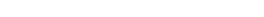in the sense that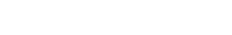If ƒ is infinitely differentiable, then this is the beginning of the Taylor series
Taylor series
In mathematics, a Taylor series is a representation of a function as an infinite sum of terms that are calculated from the values of the function's derivatives at a single point....

for ƒ.

### Inflection point

A point where the second derivative of a function changes sign is called an inflection point. At an inflection point, the second derivative may be zero, as in the case of the inflection point x=0 of the function y=x3, or it may fail to exist, as in the case of the inflection point x=0 of the function y=x1/3. At an inflection point, a function switches from being a convex function
Convex function
In mathematics, a real-valued function f defined on an interval is called convex if the graph of the function lies below the line segment joining any two points of the graph. Equivalently, a function is convex if its epigraph is a convex set...

to being a concave function
Concave function
In mathematics, a concave function is the negative of a convex function. A concave function is also synonymously called concave downwards, concave down, convex upwards, convex cap or upper convex.-Definition:...

or vice versa.

### Leibniz's notation

The notation for derivatives introduced by Gottfried Leibniz
Gottfried Leibniz
Gottfried Wilhelm Leibniz was a German philosopher and mathematician. He wrote in different languages, primarily in Latin , French and German ....

is one of the earliest. It is still commonly used when the equation y = ƒ(x) is viewed as a functional relationship between dependent and independent variables
Dependent and independent variables
The terms "dependent variable" and "independent variable" are used in similar but subtly different ways in mathematics and statistics as part of the standard terminology in those subjects...

. Then the first derivative is denoted by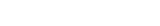and was once thought of as an infinitesimal
Infinitesimal
Infinitesimals have been used to express the idea of objects so small that there is no way to see them or to measure them. The word infinitesimal comes from a 17th century Modern Latin coinage infinitesimus, which originally referred to the "infinite-th" item in a series.In common speech, an...

quotient. Higher derivatives are expressed using the notation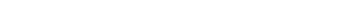for the nth derivative of y = ƒ(x) (with respect to x). These are abbreviations for multiple applications of the derivative operator. For example,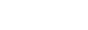With Leibniz's notation, we can write the derivative of y at the point x = a in two different ways: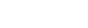Leibniz's notation allows one to specify the variable for differentiation (in the denominator). This is especially relevant for partial differentiation
Partial derivative
In mathematics, a partial derivative of a function of several variables is its derivative with respect to one of those variables, with the others held constant...

. It also makes the chain rule
Chain rule
In calculus, the chain rule is a formula for computing the derivative of the composition of two or more functions. That is, if f is a function and g is a function, then the chain rule expresses the derivative of the composite function in terms of the derivatives of f and g.In integration, the...

easy to remember: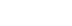### Lagrange's notation

Sometimes referred to as prime notation, one of the most common modern notations for differentiation is due to Joseph-Louis Lagrange and uses the prime mark
Prime (symbol)
The prime symbol , double prime symbol , and triple prime symbol , etc., are used to designate several different units, and for various other purposes in mathematics, the sciences and linguistics...

, so that the derivative of a function ƒ(x) is denoted ƒ′(x) or simply ƒ′. Similarly, the second and third derivatives are denoted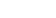and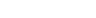To denote the number of derivatives beyond this point, some authors use Roman numerals in superscript
Subscript and superscript
A subscript or superscript is a number, figure, symbol, or indicator that appears smaller than the normal line of type and is set slightly below or above it – subscripts appear at or below the baseline, while superscripts are above...

, whereas others place the number in parentheses: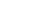or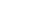The latter notation generalizes to yield the notation ƒ (n) for the nth derivative of ƒ — this notation is most useful when we wish to talk about the derivative as being a function itself, as in this case the Leibniz notation can become cumbersome.

### Newton's notation

Newton's notation for differentiation, also called the dot notation, places a dot over the function name to represent a time derivative. If y = ƒ(t), then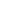and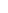denote, respectively, the first and second derivatives of y with respect to t. This notation is used exclusively for time derivative
Time derivative
A time derivative is a derivative of a function with respect to time, usually interpreted as the rate of change of the value of the function. The variable denoting time is usually written as t\,.-Notation:...

s, meaning that the independent variable of the function represents time
Time
Time is a part of the measuring system used to sequence events, to compare the durations of events and the intervals between them, and to quantify rates of change such as the motions of objects....

. It is very common in physics
Physics
Physics is a natural science that involves the study of matter and its motion through spacetime, along with related concepts such as energy and force. More broadly, it is the general analysis of nature, conducted in order to understand how the universe behaves.Physics is one of the oldest academic...

and in mathematical disciplines connected with physics such as differential equation
Differential equation
A differential equation is a mathematical equation for an unknown function of one or several variables that relates the values of the function itself and its derivatives of various orders...

s. While the notation becomes unmanageable for high-order derivatives, in practice only very few derivatives are needed.

### Euler's notation

Euler
Leonhard Euler
Leonhard Euler was a pioneering Swiss mathematician and physicist. He made important discoveries in fields as diverse as infinitesimal calculus and graph theory. He also introduced much of the modern mathematical terminology and notation, particularly for mathematical analysis, such as the notion...

's notation uses a differential operator
Differential operator
In mathematics, a differential operator is an operator defined as a function of the differentiation operator. It is helpful, as a matter of notation first, to consider differentiation as an abstract operation, accepting a function and returning another .This article considers only linear operators,...

D, which is applied to a function ƒ to give the first derivative Df. The second derivative is denoted D2ƒ, and the nth derivative is denoted Dnƒ.

If y = ƒ(x) is a dependent variable, then often the subscript x is attached to the D to clarify the independent variable x.
Euler's notation is then written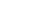or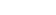,
although this subscript is often omitted when the variable x is understood, for instance when this is the only variable present in the expression.

Euler's notation is useful for stating and solving linear differential equation
Linear differential equation
Linear differential equations are of the formwhere the differential operator L is a linear operator, y is the unknown function , and the right hand side ƒ is a given function of the same nature as y...

s.

## Computing the derivative

The derivative of a function can, in principle, be computed from the definition by considering the difference quotient, and computing its limit. In practice, once the derivatives of a few simple functions are known, the derivatives of other functions are more easily computed using rules for obtaining derivatives of more complicated functions from simpler ones.

### Derivatives of elementary functions

Most derivative computations eventually require taking the derivative of some common functions. The following incomplete list gives some of the most frequently used functions of a single real variable and their derivatives.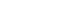where r is any real number
Real number
In mathematics, a real number is a value that represents a quantity along a continuum, such as -5 , 4/3 , 8.6 , √2 and π...

, then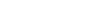wherever this function is defined. For example, if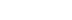, then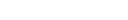and the derivative function is defined only for positive x, not for . When r = 0, this rule implies that f′(x) is zero for , which is almost the constant rule (stated below).

• Exponential
Exponential function
In mathematics, the exponential function is the function ex, where e is the number such that the function ex is its own derivative. The exponential function is used to model a relationship in which a constant change in the independent variable gives the same proportional change In mathematics,...

and logarithm
Logarithm
The logarithm of a number is the exponent by which another fixed value, the base, has to be raised to produce that number. For example, the logarithm of 1000 to base 10 is 3, because 1000 is 10 to the power 3: More generally, if x = by, then y is the logarithm of x to base b, and is written...

ic functions: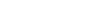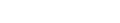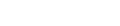• Trigonometric function
Trigonometric function
In mathematics, the trigonometric functions are functions of an angle. They are used to relate the angles of a triangle to the lengths of the sides of a triangle...

s: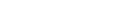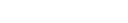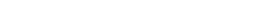• Inverse trigonometric function
Inverse trigonometric function
In mathematics, the inverse trigonometric functions are the inverse functions of the trigonometric functions with suitably restricted domains .The notations sin−1, cos−1, etc...

s: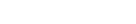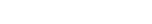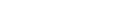### Rules for finding the derivative

In many cases, complicated limit calculations by direct application of Newton's difference quotient can be avoided using differentiation rules. Some of the most basic rules are the following.
• Constant rule: if ƒ(x) is constant, then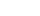• Sum rule
Linearity of differentiation
In mathematics, the linearity of differentiation is a most fundamental property of the derivative, in differential calculus. It follows from the sum rule in differentiation and the constant factor rule in differentiation...

: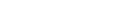for all functions ƒ and g and all real numbers a and b.
• Product rule
Product rule
In calculus, the product rule is a formula used to find the derivatives of products of two or more functions. It may be stated thus:'=f'\cdot g+f\cdot g' \,\! or in the Leibniz notation thus:...

: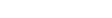for all functions ƒ and g.
• Quotient rule: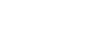for all functions ƒ and g where g ≠ 0.
• Chain rule
Chain rule
In calculus, the chain rule is a formula for computing the derivative of the composition of two or more functions. That is, if f is a function and g is a function, then the chain rule expresses the derivative of the composite function in terms of the derivatives of f and g.In integration, the...

: If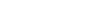, then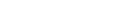### Example computation

The derivative of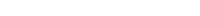is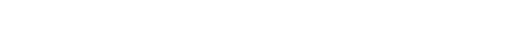Here the second term was computed using the chain rule
Chain rule
In calculus, the chain rule is a formula for computing the derivative of the composition of two or more functions. That is, if f is a function and g is a function, then the chain rule expresses the derivative of the composite function in terms of the derivatives of f and g.In integration, the...

and third using the product rule
Product rule
In calculus, the product rule is a formula used to find the derivatives of products of two or more functions. It may be stated thus:'=f'\cdot g+f\cdot g' \,\! or in the Leibniz notation thus:...

. The known derivatives of the elementary functions x2, x4, sin(x), ln(x) and exp(x) = ex, as well as the constant 7, were also used.

### Derivatives of vector valued functions

A vector-valued function
Vector-valued function
A vector-valued function also referred to as a vector function is a mathematical function of one or more variables whose range is a set of multidimensional vectors or infinite-dimensional vectors. The input of a vector-valued function could be a scalar or a vector...

y(t) of a real variable sends real numbers to vectors in some vector space
Vector space
A vector space is a mathematical structure formed by a collection of vectors: objects that may be added together and multiplied by numbers, called scalars in this context. Scalars are often taken to be real numbers, but one may also consider vector spaces with scalar multiplication by complex...

Rn. A vector-valued function can be split up into its coordinate functions y1(t), y2(t), …, yn(t), meaning that y(t) = (y1(t), ..., yn(t)). This includes, for example, parametric curves in R2 or R3. The coordinate functions are real valued functions, so the above definition of derivative applies to them. The derivative of y(t) is defined to be the vector, called the tangent vector
Differential geometry of curves
Differential geometry of curves is the branch of geometry that dealswith smooth curves in the plane and in the Euclidean space by methods of differential and integral calculus....

, whose coordinates are the derivatives of the coordinate functions. That is,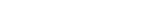Equivalently,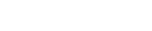if the limit exists. The subtraction in the numerator is subtraction of vectors, not scalars. If the derivative of y exists for every value of t, then y′ is another vector valued function.

If e1, …, en is the standard basis for Rn, then y(t) can also be written as y1(t)e1 + … + yn(t)en. If we assume that the derivative of a vector-valued function retains the linearity
Linearity of differentiation
In mathematics, the linearity of differentiation is a most fundamental property of the derivative, in differential calculus. It follows from the sum rule in differentiation and the constant factor rule in differentiation...

property, then the derivative of y(t) must be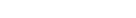because each of the basis vectors is a constant.

This generalization is useful, for example, if y(t) is the position vector of a particle at time t; then the derivative y′(t) is the velocity
Velocity
In physics, velocity is speed in a given direction. Speed describes only how fast an object is moving, whereas velocity gives both the speed and direction of the object's motion. To have a constant velocity, an object must have a constant speed and motion in a constant direction. Constant ...

vector of the particle at time t.

### Partial derivatives

Suppose that ƒ is a function that depends on more than one variable. For instance,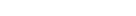ƒ can be reinterpreted as a family of functions of one variable indexed by the other variables: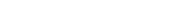In other words, every value of x chooses a function, denoted fx, which is a function of one real number. That is,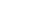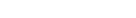Once a value of x is chosen, say a, then f(x,y) determines a function fa that sends y to a² + ay + y²: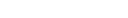In this expression, a is a constant, not a variable, so fa is a function of only one real variable. Consequently the definition of the derivative for a function of one variable applies: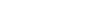The above procedure can be performed for any choice of a. Assembling the derivatives together into a function gives a function that describes the variation of ƒ in the y direction: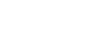This is the partial derivative of ƒ with respect to y. Here ∂ is a rounded d called the partial derivative symbol. To distinguish it from the letter d, ∂ is sometimes pronounced "der", "del", or "partial" instead of "dee".

In general, the partial derivative of a function ƒ(x1, …, xn) in the direction xi at the point (a1 …, an) is defined to be: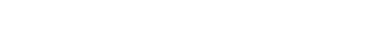In the above difference quotient, all the variables except xi are held fixed. That choice of fixed values determines a function of one variable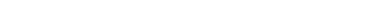and, by definition,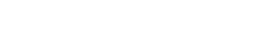In other words, the different choices of a index a family of one-variable functions just as in the example above. This expression also shows that the computation of partial derivatives reduces to the computation of one-variable derivatives.

An important example of a function of several variables is the case of a scalar-valued function ƒ(x1,...xn) on a domain in Euclidean space Rn (e.g., on R² or R³). In this case ƒ has a partial derivative ∂ƒ/∂xj with respect to each variable xj. At the point a, these partial derivatives define the vector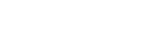This vector is called the gradient
Gradient
In vector calculus, the gradient of a scalar field is a vector field that points in the direction of the greatest rate of increase of the scalar field, and whose magnitude is the greatest rate of change....

of ƒ at a. If ƒ is differentiable at every point in some domain, then the gradient is a vector-valued function ∇ƒ that takes the point a to the vector ∇f(a). Consequently the gradient determines a vector field
Vector field
In vector calculus, a vector field is an assignmentof a vector to each point in a subset of Euclidean space. A vector field in the plane for instance can be visualized as an arrow, with a given magnitude and direction, attached to each point in the plane...

.

### Directional derivatives

If ƒ is a real-valued function on Rn, then the partial derivatives of ƒ measure its variation in the direction of the coordinate axes. For example, if ƒ is a function of x and y, then its partial derivatives measure the variation in ƒ in the x direction and the y direction. They do not, however, directly measure the variation of ƒ in any other direction, such as along the diagonal line y = x. These are measured using directional derivatives. Choose a vector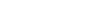The directional derivative of ƒ in the direction of v at the point x is the limit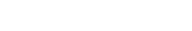In some cases it may be easier to compute or estimate the directional derivative after changing the length of the vector. Often this is done to turn the problem into the computation of a directional derivative in the direction of a unit vector. To see how this works, suppose that v = λu. Substitute h = k/λ into the difference quotient. The difference quotient becomes: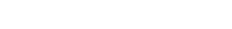This is λ times the difference quotient for the directional derivative of f with respect to u. Furthermore, taking the limit as h tends to zero is the same as taking the limit as k tends to zero because h and k are multiples of each other. Therefore Dv(ƒ) = λDu(ƒ). Because of this rescaling property, directional derivatives are frequently considered only for unit vectors.

If all the partial derivatives of ƒ exist and are continuous at x, then they determine the directional derivative of ƒ in the direction v by the formula: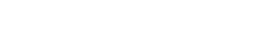This is a consequence of the definition of the total derivative
Total derivative
In the mathematical field of differential calculus, the term total derivative has a number of closely related meanings.The total derivative of a function f, of several variables, e.g., t, x, y, etc., with respect to one of its input variables, e.g., t, is different from the partial derivative...

. It follows that the directional derivative is linear in v, meaning that Dv + w(ƒ) = Dv(ƒ) + Dw(ƒ).

The same definition also works when ƒ is a function with values in Rm. The above definition is applied to each component of the vectors. In this case, the directional derivative is a vector in Rm.

### The total derivative, the total differential and the Jacobian

When ƒ is a function from an open subset of Rn to Rm, then the directional derivative of ƒ in a chosen direction is the best linear approximation to ƒ at that point and in that direction. But when , no single directional derivative can give a complete picture of the behavior of ƒ. The total derivative, also called the (total) differential
Differential (calculus)
In calculus, a differential is traditionally an infinitesimally small change in a variable. For example, if x is a variable, then a change in the value of x is often denoted Δx . The differential dx represents such a change, but is infinitely small...

, gives a complete picture by considering all directions at once. That is, for any vector v starting at a, the linear approximation formula holds: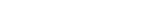Just like the single-variable derivative, is chosen so that the error in this approximation is as small as possible.

If n and m are both one, then the derivative is a number and the expression is the product of two numbers. But in higher dimensions, it is impossible for to be a number. If it were a number, then would be a vector in Rn while the other terms would be vectors in Rm, and therefore the formula would not make sense. For the linear approximation formula to make sense, must be a function that sends vectors in Rn to vectors in Rm, and must denote this function evaluated at v.

To determine what kind of function it is, notice that the linear approximation formula can be rewritten as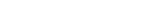Notice that if we choose another vector w, then this approximate equation determines another approximate equation by substituting w for v. It determines a third approximate equation by substituting both w for v and for a. By subtracting these two new equations, we get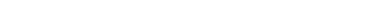If we assume that v is small and that the derivative varies continuously in a, then is approximately equal to , and therefore the right-hand side is approximately zero. The left-hand side can be rewritten in a different way using the linear approximation formula with substituted for v. The linear approximation formula implies: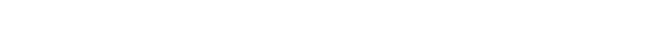This suggests that is a linear transformation
Linear transformation
In mathematics, a linear map, linear mapping, linear transformation, or linear operator is a function between two vector spaces that preserves the operations of vector addition and scalar multiplication. As a result, it always maps straight lines to straight lines or 0...

from the vector space Rn to the vector space Rm. In fact, it is possible to make this a precise derivation by measuring the error in the approximations. Assume that the error in these linear approximation formula is bounded by a constant times ||v||, where the constant is independent of v but depends continuously on a. Then, after adding an appropriate error term, all of the above approximate equalities can be rephrased as inequalities. In particular, is a linear transformation up to a small error term. In the limit as v and w tend to zero, it must therefore be a linear transformation. Since we define the total derivative by taking a limit as v goes to zero, must be a linear transformation.

In one variable, the fact that the derivative is the best linear approximation is expressed by the fact that it is the limit of difference quotients. However, the usual difference quotient does not make sense in higher dimensions because it is not usually possible to divide vectors. In particular, the numerator and denominator of the difference quotient are not even in the same vector space: The numerator lies in the codomain Rm while the denominator lies in the domain Rn. Furthermore, the derivative is a linear transformation, a different type of object from both the numerator and denominator. To make precise the idea that is the best linear approximation, it is necessary to adapt a different formula for the one-variable derivative in which these problems disappear. If , then the usual definition of the derivative may be manipulated to show that the derivative of ƒ at a is the unique number such that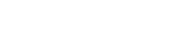This is equivalent to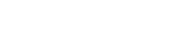because the limit of a function tends to zero if and only if the limit of the absolute value of the function tends to zero. This last formula can be adapted to the many-variable situation by replacing the absolute values with norm
Norm (mathematics)
In linear algebra, functional analysis and related areas of mathematics, a norm is a function that assigns a strictly positive length or size to all vectors in a vector space, other than the zero vector...

s.

The definition of the total derivative of ƒ at a, therefore, is that it is the unique linear transformation such that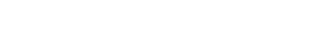Here h is a vector in Rn, so the norm in the denominator is the standard length on Rn. However, ƒ′(a)h is a vector in Rm, and the norm in the numerator is the standard length on Rm. If v is a vector starting at a, then is called the pushforward of v by ƒ and is sometimes written .

If the total derivative exists at a, then all the partial derivatives and directional derivatives of ƒ exist at a, and for all v, is the directional derivative of ƒ in the direction v. If we write ƒ using coordinate functions, so that ƒ = (ƒ1, ƒ2, ..., ƒm), then the total derivative can be expressed using the partial derivatives as a matrix
Matrix (mathematics)
In mathematics, a matrix is a rectangular array of numbers, symbols, or expressions. The individual items in a matrix are called its elements or entries. An example of a matrix with six elements isMatrices of the same size can be added or subtracted element by element...

. This matrix is called the Jacobian matrix of ƒ at a: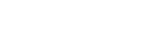The existence of the total derivative ƒ′(a) is strictly stronger than the existence of all the partial derivatives, but if the partial derivatives exist and are continuous, then the total derivative exists, is given by the Jacobian, and depends continuously on a.

The definition of the total derivative subsumes the definition of the derivative in one variable. That is, if ƒ is a real-valued function of a real variable, then the total derivative exists if and only if the usual derivative exists. The Jacobian matrix reduces to a 1×1 matrix whose only entry is the derivative ƒ′(x). This 1×1 matrix satisfies the property that is approximately zero, in other words that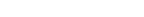Up to changing variables, this is the statement that the function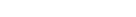is the best linear approximation to ƒ at a.

The total derivative of a function does not give another function in the same way as the one-variable case. This is because the total derivative of a multivariable function has to record much more information than the derivative of a single-variable function. Instead, the total derivative gives a function from the tangent bundle
Tangent bundle
In differential geometry, the tangent bundle of a differentiable manifold M is the disjoint unionThe disjoint union assures that for any two points x1 and x2 of manifold M the tangent spaces T1 and T2 have no common vector...

of the source to the tangent bundle of the target.

The natural analog of second, third, and higher-order total derivatives is not a linear transformation, is not a function on the tangent bundle, and is not built by repeatedly taking the total derivative. The analog of a higher-order derivative, called a jet
Jet (mathematics)
In mathematics, the jet is an operation which takes a differentiable function f and produces a polynomial, the truncated Taylor polynomial of f, at each point of its domain...

, cannot be a linear transformation because higher-order derivatives reflect subtle geometric information, such as concavity, which cannot be described in terms of linear data such as vectors. It cannot be a function on the tangent bundle because the tangent bundle only has room for the base space and the directional derivatives. Because jets capture higher-order information, they take as arguments additional coordinates representing higher-order changes in direction. The space determined by these additional coordinates is called the jet bundle
Jet bundle
In differential geometry, the jet bundle is a certain construction which makes a new smooth fiber bundle out of a given smooth fiber bundle. It makes it possible to write differential equations on sections of a fiber bundle in an invariant form...

. The relation between the total derivative and the partial derivatives of a function is paralleled in the relation between the kth order jet of a function and its partial derivatives of order less than or equal to k.

## Generalizations

The concept of a derivative can be extended to many other settings. The common thread is that the derivative of a function at a point serves as a linear approximation
Linear approximation
In mathematics, a linear approximation is an approximation of a general function using a linear function . They are widely used in the method of finite differences to produce first order methods for solving or approximating solutions to equations.-Definition:Given a twice continuously...

of the function at that point.
• An important generalization of the derivative concerns complex functions of complex variable
Complex number
A complex number is a number consisting of a real part and an imaginary part. Complex numbers extend the idea of the one-dimensional number line to the two-dimensional complex plane by using the number line for the real part and adding a vertical axis to plot the imaginary part...

s, such as functions from (a domain in) the complex numbers C to C. The notion of the derivative of such a function is obtained by replacing real variables with complex variables in the definition. If C is identified with R² by writing a complex number z as x + i y, then a differentiable function from C to C is certainly differentiable as a function from R² to R² (in the sense that its partial derivatives all exist), but the converse is not true in general: the complex derivative only exists if the real derivative is complex linear and this imposes relations between the partial derivatives called the Cauchy Riemann equations — see holomorphic function
Holomorphic function
In mathematics, holomorphic functions are the central objects of study in complex analysis. A holomorphic function is a complex-valued function of one or more complex variables that is complex differentiable in a neighborhood of every point in its domain...

s.
• Another generalization concerns functions between differentiable or smooth manifolds. Intuitively speaking such a manifold M is a space that can be approximated near each point x by a vector space called its tangent space
Tangent space
In mathematics, the tangent space of a manifold facilitates the generalization of vectors from affine spaces to general manifolds, since in the latter case one cannot simply subtract two points to obtain a vector pointing from one to the other....

: the prototypical example is a smooth surface in R³. The derivative (or differential) of a (differentiable) map ƒ: M → N between manifolds, at a point x in M, is then a linear map from the tangent space of M at x to the tangent space of N at ƒ(x). The derivative function becomes a map between the tangent bundle
Tangent bundle
In differential geometry, the tangent bundle of a differentiable manifold M is the disjoint unionThe disjoint union assures that for any two points x1 and x2 of manifold M the tangent spaces T1 and T2 have no common vector...

s of M and N. This definition is fundamental in differential geometry and has many uses — see pushforward (differential) and pullback (differential geometry).
• Differentiation can also be defined for maps between infinite dimensional
Dimension (vector space)
In mathematics, the dimension of a vector space V is the cardinality of a basis of V. It is sometimes called Hamel dimension or algebraic dimension to distinguish it from other types of dimension...

vector space
Vector space
A vector space is a mathematical structure formed by a collection of vectors: objects that may be added together and multiplied by numbers, called scalars in this context. Scalars are often taken to be real numbers, but one may also consider vector spaces with scalar multiplication by complex...

s such as Banach space
Banach space
In mathematics, Banach spaces is the name for complete normed vector spaces, one of the central objects of study in functional analysis. A complete normed vector space is a vector space V with a norm ||·|| such that every Cauchy sequence in V has a limit in V In mathematics, Banach spaces is the...

s and Fréchet space
Fréchet space
In functional analysis and related areas of mathematics, Fréchet spaces, named after Maurice Fréchet, are special topological vector spaces. They are generalizations of Banach spaces...

s. There is a generalization both of the directional derivative, called the Gâteaux derivative
Gâteaux derivative
In mathematics, the Gâteaux differential or Gâteaux derivative is a generalization of the concept of directional derivative in differential calculus. Named after René Gâteaux, a French mathematician who died young in World War I, it is defined for functions between locally convex topological vector...

, and of the differential, called the Fréchet derivative
Fréchet derivative
In mathematics, the Fréchet derivative is a derivative defined on Banach spaces. Named after Maurice Fréchet, it is commonly used to formalize the concept of the functional derivative used widely in the calculus of variations. Intuitively, it generalizes the idea of linear approximation from...

.
• One deficiency of the classical derivative is that not very many functions are differentiable. Nevertheless, there is a way of extending the notion of the derivative so that all continuous
Continuous function
In mathematics, a continuous function is a function for which, intuitively, "small" changes in the input result in "small" changes in the output. Otherwise, a function is said to be "discontinuous". A continuous function with a continuous inverse function is called "bicontinuous".Continuity of...

functions and many other functions can be differentiated using a concept known as the weak derivative
Weak derivative
In mathematics, a weak derivative is a generalization of the concept of the derivative of a function for functions not assumed differentiable, but only integrable, i.e. to lie in the Lebesgue space L^1. See distributions for an even more general definition.- Definition :Let u be a function in the...

. The idea is to embed the continuous functions in a larger space called the space of distributions
Distribution (mathematics)
In mathematical analysis, distributions are objects that generalize functions. Distributions make it possible to differentiate functions whose derivatives do not exist in the classical sense. In particular, any locally integrable function has a distributional derivative...

and only require that a function is differentiable "on average".
• The properties of the derivative have inspired the introduction and study of many similar objects in algebra and topology — see, for example, differential algebra
Differential algebra
In mathematics, differential rings, differential fields, and differential algebras are rings, fields, and algebras equipped with a derivation, which is a unary function that is linear and satisfies the Leibniz product law...

.
• The discrete equivalent of differentiation is finite difference
Finite difference
A finite difference is a mathematical expression of the form f − f. If a finite difference is divided by b − a, one gets a difference quotient...

s. The study of differential calculus is unified with the calculus of finite differences in time scale calculus
Time scale calculus
In mathematics, time-scale calculus is a unification of the theory of difference equations with that of differential equations, unifying integral and differential calculus with the calculus of finite differences, offering a formalism for studying hybrid discrete–continuous dynamical systems...

.
• Also see arithmetic derivative
Arithmetic derivative
In number theory, the arithmetic derivative, or number derivative, is a function defined for integers, based on prime factorization, by analogy with the product rule for the derivative of a function that is used in mathematical analysis.-Definition:...

.

## See also

• Applications of derivatives
• Automatic differentiation
Automatic differentiation
In mathematics and computer algebra, automatic differentiation , sometimes alternatively called algorithmic differentiation, is a set of techniques to numerically evaluate the derivative of a function specified by a computer program...

• Differentiability class
• Differintegral
• Generalizations of the derivative
• Integral
Integral
Integration is an important concept in mathematics and, together with its inverse, differentiation, is one of the two main operations in calculus...

• Linearization
Linearization
In mathematics and its applications, linearization refers to finding the linear approximation to a function at a given point. In the study of dynamical systems, linearization is a method for assessing the local stability of an equilibrium point of a system of nonlinear differential equations or...

• Multiplicative inverse
Multiplicative inverse
In mathematics, a multiplicative inverse or reciprocal for a number x, denoted by 1/x or x−1, is a number which when multiplied by x yields the multiplicative identity, 1. The multiplicative inverse of a fraction a/b is b/a. For the multiplicative inverse of a real number, divide 1 by the...

• Numerical differentiation
Numerical differentiation
In numerical analysis, numerical differentiation describes algorithms for estimating the derivative of a mathematical function or function subroutine using values of the function and perhaps other knowledge about the function.-Finite difference formulae:...

• Symmetric derivative
• Differentiation rules
Differentiation rules
This article is a summary of the differentiation rules in elementary calculus, i.e. rules for computing the derivative of a function in calculus and mathematical analysis.-Nomenclature:...

### Web pages

The source of this article is wikipedia, the free encyclopedia.  The text of this article is licensed under the GFDL.